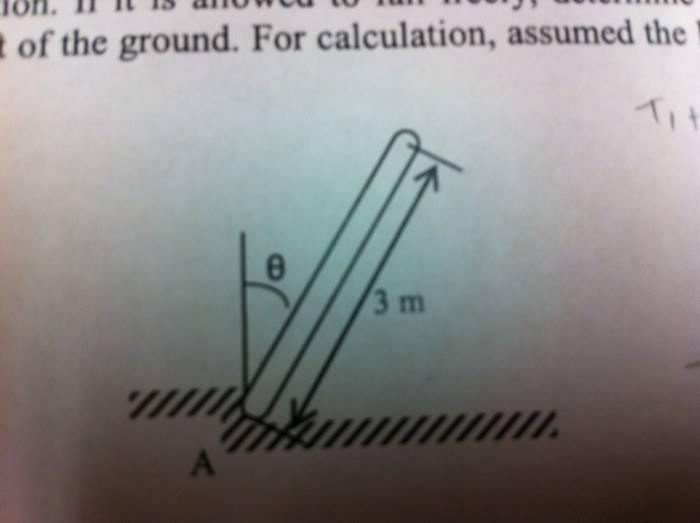# Conservation of Energy falling rod

## Homework Statement

A uniform ladder having mass 14kg and length 3m is released from rest when it is in the vertical position. If it is allowed to fall freely, determine the angle Theta at which the bottom end A starts to lift off the ground. For calculation assume the ladder is a slender rod and neglect friction at A.## Homework Equations

T_1+V_1=T_2+V_2[/B]

## The Attempt at a Solution

T_1=0

V_1=mgh
=mgL/2

T_2=1/2I(omega)^2
where I for end of rod = 1/3mL^2

so 1/2(1/3mL^2)(omega)^2

V_2=mgh
=mgL/2cos(Theta)

Solve T_1+V_1=T_2+V_2

g(1-cos(Theta))=(omega)^2

I think we now need solve for the forces at point A, but I dont know how...

I also don't really understand at what point A would "lift"

Any help is appreciated!

Thanks[/B]